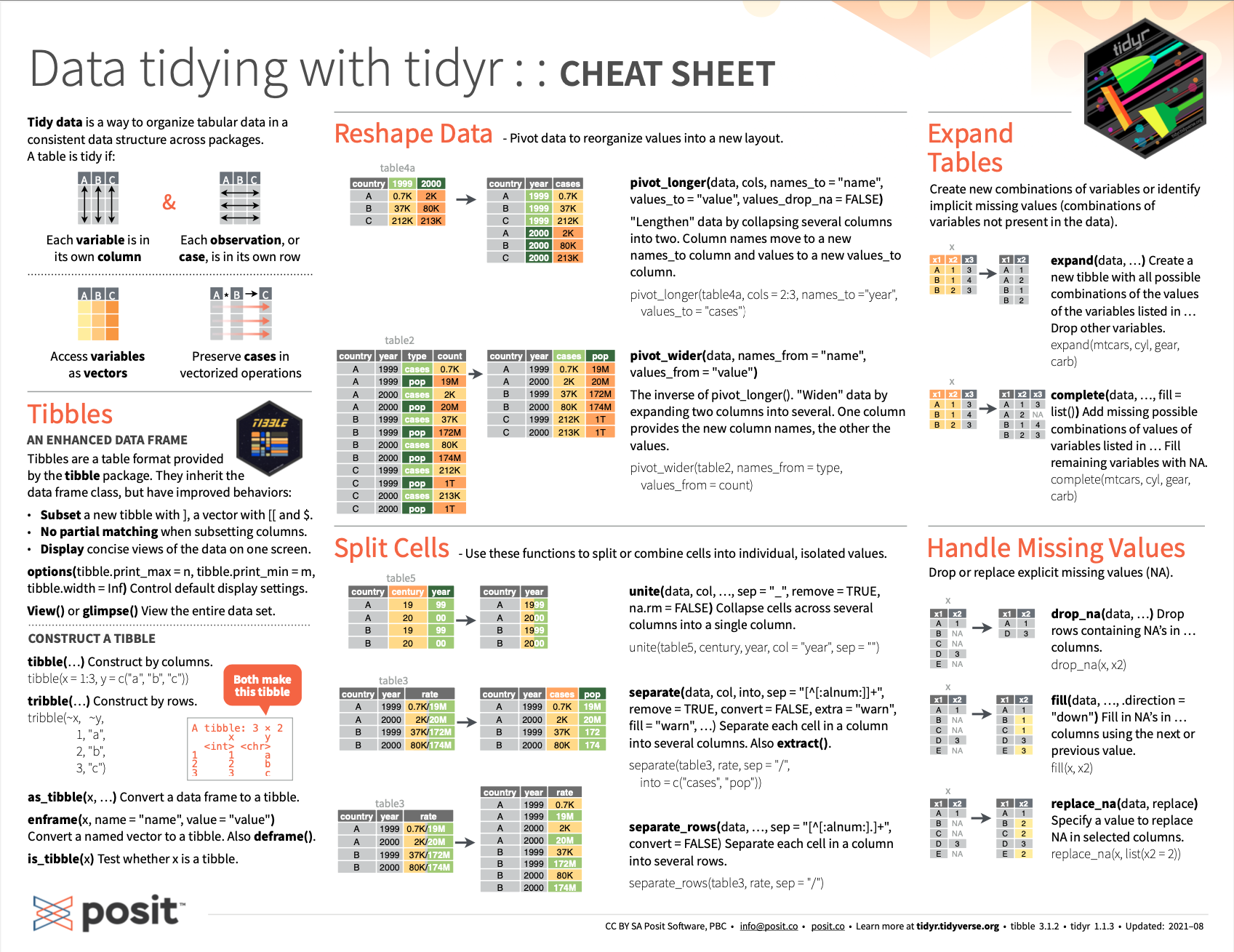## 5.3 SummaryTibbles are simple data tables and the primary way of representing data (in rows of cases and columns of variables) in the tidyverse . In this chapter, we examined essential commands of the tibble package :

1. as_tibble() converts (or coerces) an existing rectangle of data (e.g., a data frame or matrix) into a tibble.

2. tibble() converts several vectors into (the columns of) a tibble.

3. tribble() converts a table (entered row-by-row) into a tibble.

Thus, the three functions differ by the types of inputs they expect, but have in common that they create a tibble as their output.

While some R veterans may prefer data frames, tibbles are becoming increasingly popular. But if we ever need to transform a tibble tb into a data frame, we can always use as.data.frame(tb).

By working through this chapter, you are now able to use tibble commands to:

1. turn rectangular data into a tibble;
2. turn vectors of variables into (the columns of) a tibble;
3. turn cases into (the rows of) a tibble;
4. enter some data into a tibble; and
5. understand some limitations of rectangular data.

The relation between tibbles and data frames and key tibble commands are summarized on the back of the RStudio cheatsheet on Data Import:Figure 5.2: Essential tibble commands on the back of the RStudio cheatsheet on Data Import.

Let’s test our knowledge and skills concerning tibbles by completing the following exercises.

### References

Müller, K., & Wickham, H. (2020). tibble: Simple data frames. Retrieved from https://CRAN.R-project.org/package=tibble
Wickham, H. (2019c). tidyverse: Easily install and load the ’tidyverse’. Retrieved from https://CRAN.R-project.org/package=tidyverse